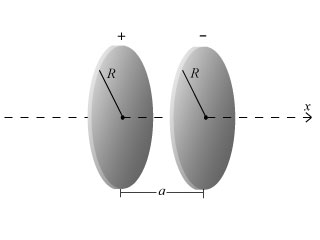# Problem: Consider two thin disks, of negligible thickness, of radius R oriented perpendicular to the x axis such that the x axis runs through the center of each disk. The disk centered at x = 0 has positive charge density η, and the disk centered at x = a has negative charge density –η, where the charge density is charge per unit area.(a) What is the magnitude E of the electric field at the point on the x-axis with x coordinate a/2?(b) For what value of the ratio R/a of plate radius to separation between the plates does the electric field at the point x = a/2 on the x axis differ by 1% from the result n/ε0 for infinite sheets?

###### FREE Expert Solution

In this problem, we'll carry out some calculations based on the scenario given.

The expression for the electric field on a point on the x-axis is:

$\overline{){\mathbf{E}}{\mathbf{=}}\frac{\mathbf{x}\mathbf{q}}{\mathbf{4}\mathbf{\pi }{\mathbf{\epsilon }}_{\mathbf{0}}\mathbf{\left(}{\mathbf{R}}^{\mathbf{2}}\mathbf{+}{\mathbf{x}}^{\mathbf{2}}\mathbf{\right)}}}$

97% (210 ratings)###### Problem Details

Consider two thin disks, of negligible thickness, of radius R oriented perpendicular to the x axis such that the x axis runs through the center of each disk. The disk centered at x = 0 has positive charge density η, and the disk centered at x = a has negative charge density –η, where the charge density is charge per unit area.(a) What is the magnitude E of the electric field at the point on the x-axis with x coordinate a/2?

(b) For what value of the ratio R/a of plate radius to separation between the plates does the electric field at the point x = a/2 on the x axis differ by 1% from the result n/ε0 for infinite sheets?